# Surface area - 6th grade (11y) - math problems

#### Number of problems found: 77

• A butterA butter cube with an edge 6.5 cm long is packed in a package with dimensions a = 28 cm, b = 15 cm. Calculate how many cm2 the package is larger than the surface of the cube.
• Empty aquariumHow much does an empty aquarium weigh with dimensions: length = 40 cm, width = 30 cm, height = 20 cm, if 1 dm2 of glass weighs 300 g? Calculate its weight in kilograms.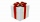How much wrapping paper is needed to wrap a cube-shaped gift with edge measuring 15 cm?
• Cloth / textileWe have cloth measure 16 square meters. How many 20 cm by 20 cm by 8 cm bags you can make? Assume bag is a cuboid without one top base.
• The cubeThe cube has a surface of 600 cm2. What is its volume?
• FlowerpotThe block-shaped flowerpot has external dimensions: length 1.25 m, width 10 cm, and height 11 cm. The thickness of the boards from which it is made is 0.8 cm. How many liters of soil is needed to fill it 1 cm below the top edge? What surface do we have to
• Calculate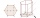Calculate the surface of a regular eleven-sided prism; if the area of its base is 58 cm2, the edge of the base is 6cm long, the height of the prism is 21 cm.
• Edge cFind the edge c of cuboid if an edge a = 20 mm, b = 30 mm and surface area S = 8000 mm2.
• Cube V2SThe volume of the cube is 27 dm cubic. Calculate the surface of the cube.
• Hexagonal prism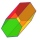Calculate the volume and surface of a regular hexagonal prism with the edge of the base a = 6 cm with the corresponding height v1 = 5.2cm and the height of the prism h = 1 dm.
• Surface and volumeCalculate the surface and volume of a cylinder whose height is 8 dm and the radius of the base circle is 2 dm
• Milk cartonsHow much paper do we need for 12 tetra packs with dimensions 6 cm, 11 cm, and 20 cm? Will 1 liter of milk fit in the box?
• Water reservoir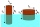What is the weight of a metal reservoir - cylinder with a diameter of 2 m and a length 8 m, if 1 m2 of sheet metal weighs 100 kg?
• Four prismsQuestion No. 1: The prism has the dimensions a = 2.5 cm, b = 100 mm, c = 12 cm. What is its volume? a) 3000 cm2 b) 300 cm2 c) 3000 cm3 d) 300 cm3 Question No.2: The prism base is a rhombus with a side length of 30 cm and a height of 27 cm. The height of t
• Two walls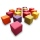Calculate the surface area of a cube in m2 if you know that the area of its two walls is 72 dm2.
• Cuboid to cubeA cuboid with dimensions of 9 cm, 6 cm, and 4 cm has the same volume as a cube. Calculate the surface of this cube.
• Surface and volumeFind the surface and volume of a cuboid whose dimensions are 1 m, 50 cm, and 6 dm.
• Volume and surface areaFind the volume and surface of a wooden block with dimensions: a = 8 cm, b = 10 cm, c = 16 cm.
• Net of a cube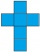We can fold this net to form a cube with a side length of 20 units. What is the surface area of the cube in square units?
• Winch drum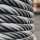Originally an empty winch drum with a diameter of 20 cm and a width of 30 cm on the rescue car, he started winding a rope with a thickness of 1 cm beautifully from edge to edge. The winch stopped after 80 turns. It remains to spin 3.54m of rope (without h

Do you have an exciting math question or word problem that you can't solve? Ask a question or post a math problem, and we can try to solve it.

We will send a solution to your e-mail address. Solved examples are also published here. Please enter the e-mail correctly and check whether you don't have a full mailbox.

Please do not submit problems from current active competitions such as Mathematical Olympiad, correspondence seminars etc...

Examples for the calculation of the surface area of ​​the solid object . Examples for 6th grade (the sixth graders).